# Redheffer's matrix

The Redheffer matrix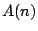is an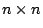matrix of 0's and 1's defined by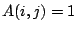if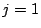or if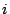divides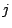, and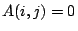otherwise. Redheffer proved thathas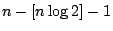eigenvalues equal to 1. Also, A has a real eigenvalue (the spectral radius) which is approximately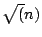, a negative eigenvalue which is approximately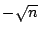and the remaining eigenvalues are small. The Riemann hypothesis is true if and only if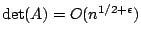for every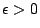.

Barrett Forcade, Rodney, and Pollington [ MR 89j:15029] give an easy proof of Redheffer's theorem. They also prove that the spectral radius ofis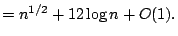See also the paper of Roesleren [ MR 87i:11111].

Vaughan [ MR 94b:11086] and [ MR 96m:11073] determines the dominant eigenvalues with an error term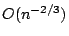and shows that the nontrivial eigenvalues are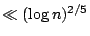(unconditionally), and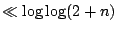on Riemann's hypothesis.

It is possible that the nontrivial eivenvalues lie in the unit disc.

Back to the main index for The Riemann Hypothesis.